AP State Syllabus AP Board 8th Class Maths Solutions Chapter 15 Playing with Numbers Ex 15.4 Textbook Questions and Answers.

## AP State Syllabus 8th Class Maths Solutions 15th Lesson Playing with Numbers Exercise 15.4Question 1.
Check whether 25110 is divisible by 45.
Solution:
The given number = 25110
If 25110 is divisible by 45 then it should be divisible by 5 and 9.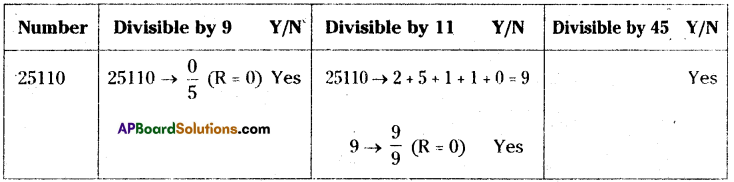∴ The number 25110 is divisible by 45Question 2.
Check whether 61479 is divisible by 81.
Solution:
If 61479 is divisible by 81 then it is divisible by 9.
If the sum of the digits of a number is dívisible by 9 then the entire number is divisible by 9.
∴ 61479 → 6 + 1 + 4 + 7 + 9 → $$\frac { 27 }{ 9 }$$ (R = 0)
∴ 61479 is divisible by 81. [∵ 9 is factor of 81]

Question 3.
Check whether 864 is divisible by 36? Verif,’ whether 864 is divisible by all the factors of 36 ?
Solution:
864 is divisible by 2 and 3.
∴ 864 is divisible by 6.
∴ 864 is divisible by 36 [ ∵ 6 is the factor of 36]
∴ Factors of 36 = 1, 2, 3, 4, 6, 9, 12, 18. 36.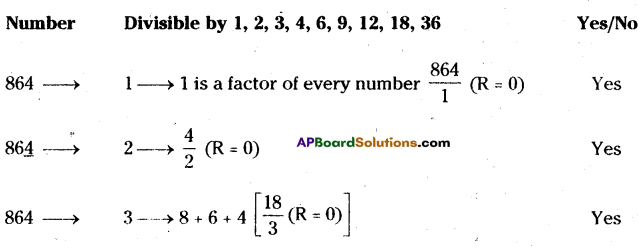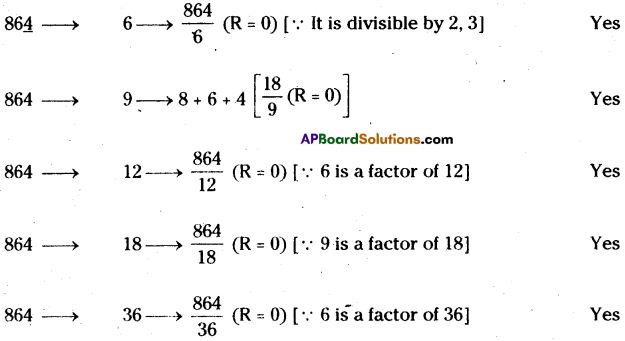∴ 864 is divisible by all the factor of 36.Question 4.
Check whether 756 is divisible by 42? Verify whether 756 is divisible by all the factors of 42?
Solution:
756 is divisible by 2 and 3.
∴ 756 is divisible by 6.
2a + 3b + c = 2 x 7 + 3 x 5 + 6 = 14 + 15 + 6 → $$\frac { 35 }{ 7 }$$ (R = 0)
∴ 756 is divisible by 7.
∴ 756 is divisible by 42. [ ∵ 6, 7 are the factors of 42]
Factors of 42 = 1, 2, 3, 6, 7, 14, 21, 42.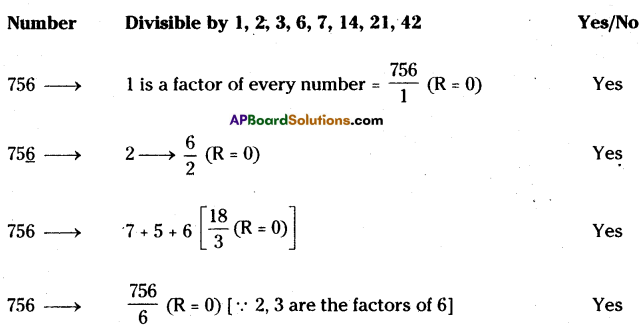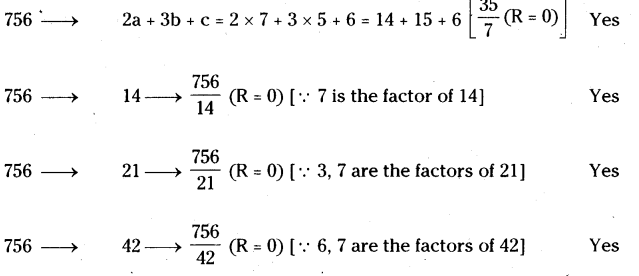∴ 756 is divisible by all the factor of 42.Question 5.
Check whether 2156 is divisible by 11 and 7? Verify whether 2156 is divisible by product of 11 and 7?
Solution: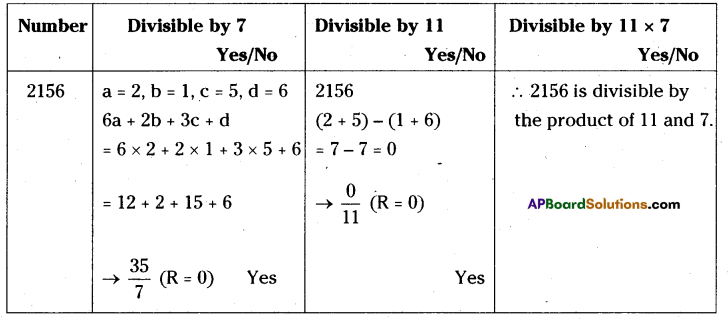Question 6.
Check whether 1435 is divisible by 5 and 7? Verify if 1435 is divisible by the product of 5 and 7?
Solution: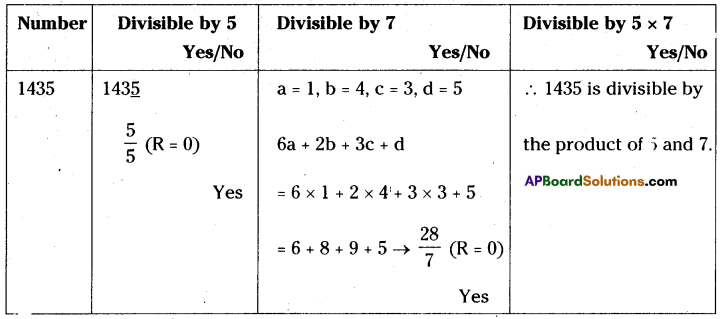Question 7.
Check whether 456 and 618 are divisible by 6’? Also check whether 6 divides the sum of 456 and 618 ‘?
Solution: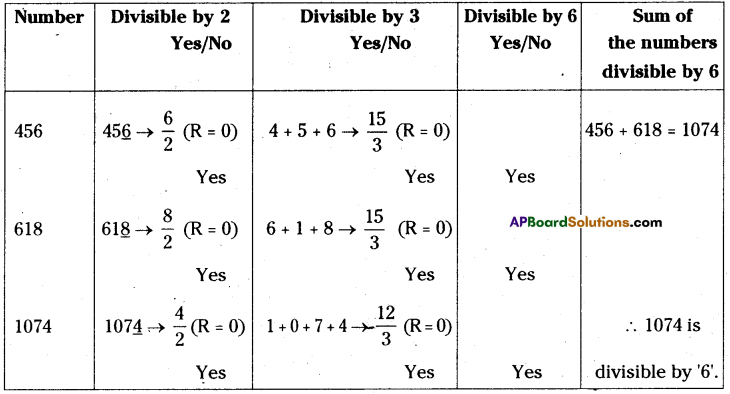Question 8.
Check whether 876 and 345 are divisible by 3. Also check whether 3 divides the difference of 876 and 345?
Solution:

 Number Divisible by 3 Y/N Difference is divisible by 3 Y/N 876 8 + 7 + 6 → $$\frac { 21 }{ 3 }$$ (R = 0) Yes 876 – 345 = 531 345 3 + 4 + 5 → $$\frac { 12 }{ 3 }$$ (R = 0) Yes The difference of 876, 345 is divisible by 3. 531 5 + 3 + 1 → $$\frac { 9 }{ 3 }$$ (R = 0) Yes

Question 9.
Check whether 22 + 23+24 is divisible by 2 or 4 or by both 2 and 4’?
Solution: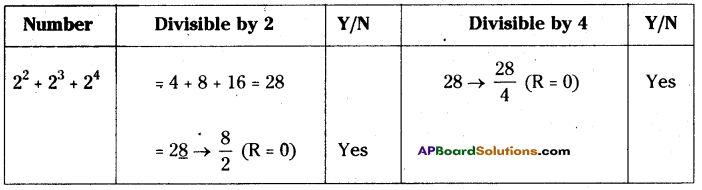∴ 22 + 23+24 is divisible by both 2 and 4.

Question 10.
Check whether 322 is divisible by 4 or 8 or by both 4 and 8’?
Solution: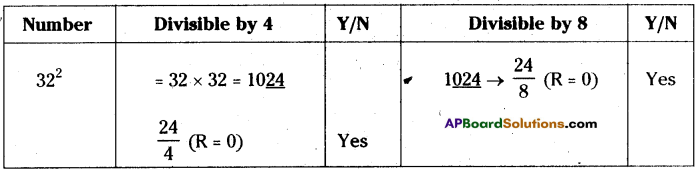322 is divisible by 4 and 8Question 11.
If A679B is a 5-dit number is divisible by 72 find ‘A’ and ‘B”?
Solution:
If A679B is divisible by 72 then it should be divisible by 8 and 9.
[ ∵ 8, 9 are the factors of 72]
A679B is divisible by 9 then
A + 6 + 7 + 9 + B = A + B + 22 = 27 (= 9 x 3)
=A + B = 5 ……………. (1)
A679B → $$\frac{79 \mathrm{~B}}{8}$$ [From B (2,4,6,8) we take B = 2]
= $$\frac{792}{8}$$ (R = 0)
∴ B = 2
From (1) ⇒ A + 2 = 5
∴ A = 3, B = 2